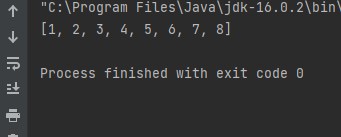### 归并排序：

#### 排序原理：

1、尽可能的一组数据拆分成两个元素相等的子组，并对每一个子组继续拆分，知道拆分后的每个子组的元素个数是1为止。

2、将相邻的两个子组进行合并成一个有序的大组

3、不断重复步骤2，直到最终只有一个组为止

#### 代码实现：

package demo02.sort;

//排序代码
public class Merge {
private static Comparable[] assist;//归并所需要的辅助数组

/*
对数组a中的元素进行排序
*/
public static void sort(Comparable[] a) {
assist = new Comparable[a.length];
int lo = 0;
int hi = a.length-1;
sort(a, lo, hi);
}

/*
对数组a中从lo到hi的元素进行排序
*/
private static void sort(Comparable[] a, int lo, int hi) {
if (hi <= lo) {
return;
}

int mid = lo + (hi - lo) / 2;

//对lo到mid之间的元素进行排序；
sort(a, lo, mid);
//对mid+1到hi之间的元素进行排序；
sort(a, mid+1, hi);
//对lo到mid这组数据和mid到hi这组数据进行归并
merge(a, lo, mid, hi);
}

/*
对数组中，从lo到mid为一组，从mid+1到hi为一组，对这两组数据进行归并
*/
private static void merge(Comparable[] a, int lo, int mid, int hi) {
//lo到mid这组数据和mid+1到hi这组数据归并到辅助数组assist对应的索引处
int i = lo;//定义一个指针，指向assist数组中开始填充数据的索引
int p1 = lo;//定义一个指针，指向第一组数据的第一个元素
int p2 = mid + 1;//定义一个指针，指向第二组数据的第一个元素

//比较左边小组和右边小组中的元素大小，哪个小，就把哪个数据填充到assist数组中
while (p1 <= mid && p2 <= hi) {
if (less(a[p1], a[p2])) {
assist[i++] = a[p1++];
} else {
assist[i++] = a[p2++];
}
}

//上面的循环结束后，如果退出循环的条件是p1<=mid，则证明左边小组中的数据已经归并完毕，如果退出循环的条件是p2<=hi,则证明右边小组的数据已经填充完毕；
//所以需要把未填充完毕的数据继续填充到assist中,//下面两个循环，只会执行其中的一个
while(p1<=mid){
assist[i++]=a[p1++];
}
while(p2<=hi){
assist[i++]=a[p2++];
}

//到现在为止，assist数组中，从lo到hi的元素是有序的，再把数据拷贝到a数组中对应的索引处

for (int index=lo;index<=hi;index++){
a[index]=assist[index];
}
}

/*
比较v元素是否小于w元素
*/
private static boolean less(Comparable v, Comparable w) {
return v.compareTo(w) < 0;
}

/*
数组元素i和j交换位置
*/
private static void exch(Comparable[] a, int i, int j) {
Comparable t = a[i];
a[i] = a[j];
a[j] = t;
}
}

package demo02.test;
import demo02.sort.Merge;
import java.util.Arrays;
public class TestMerge {
public static void main(String[] args) throws Exception {
Integer[] arr = {8, 4, 5, 7, 1, 3, 6, 2};
Merge.sort(arr);
System.out.println(Arrays.toString(arr));

}
}



#### 运行结果：Q.E.D.